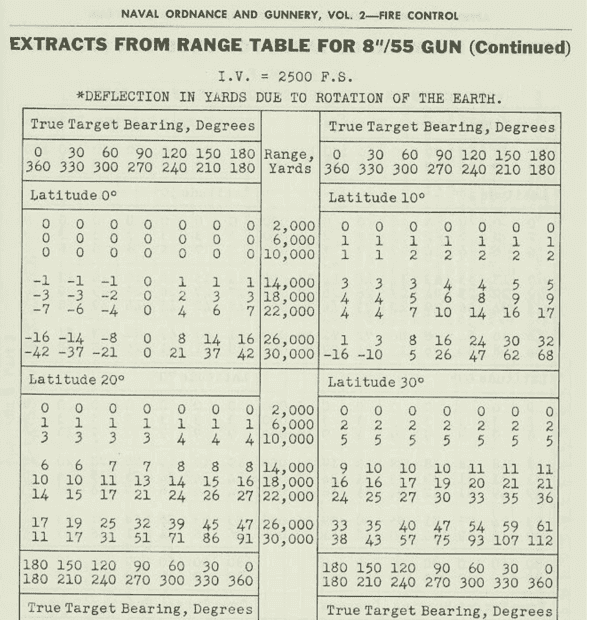# Coriolis acceleration of a projectile launched at the equator

• I
jk185
I have a question about the Coriolis acceleration experienced by a projectile launched from the surface of a rotating body.

Say a ball is launched at 45 degree angle relative to the surface at some initial velocity v0. Let's further specify that the ball is launched due north from the equator (i.e. latitude = 0). I want to calculate the Coriolis acceleration (and its x, y, z components) that is experienced by the ball. Since the launch occurs at the equator, most would say that the Coriolis acceleration is zero, but is this true? Isn't the Coriolis acceleration only 0 at the equator since the launch angle is assumed to be 0 (i.e. the motion is parallel to the surface), meaning the velocity vector is parallel to the rotational vector? If a ball is launched at some angle relative the surface, the velocity vector is no longer parallel to the surface, meaning that there should actually be a Coriolis acceleration that is experienced by the ball.

Also, I am particularly interested in long-range ballistic travel.

Is there something that I am missing here? I have looked everywhere for an answer to this question but haven't found anything that specifically addresses this question.

Any help is appreciated here. Thanks!

Homework Helper
Gold Member
•jk185
jk185
This is not exactly what I was looking for, but thank you anyways.

Mentor
Isn't the Coriolis acceleration only 0 at the equator since the launch angle is assumed to be 0 (i.e. the motion is parallel to the surface), meaning the velocity vector is parallel to the rotational vector? If a ball is launched at some angle relative the surface, the velocity vector is no longer parallel to the surface, meaning that there should actually be a Coriolis acceleration that is experienced by the ball.
That seems to be what the standard formula for calculating the Coriolis acceleration ##a_C=2\vec{\Omega}\times\vec{v}## says, yes.

•jk185•